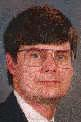html> Electronic Journal of Differential Equations Electronic Journal of Differential Equations 15th annual Conference of Applied Mathematics, Univ. of Central Oklahoma,
Electron. J. Diff. Eqns., Conf. 02, 1999, pp. 1-9.

### Fifth-order Runge-Kutta with higher order derivative approximations David Goeken & Olin Johnson

Abstract:
Given y'=f(y), standard Runge-Kutta methods perform multiple evaluations of f(y) in each integration sub-interval as required for a given accuracy. Evaluations of y''=f_yf or higher derivatives are not considered due to the assumption that the calculations involved in these functions exceed those of f. However, y'' can be approximated to sufficient accuracy from past and current evaluations of f to achieve a higher order of accuracy than is available through current functional evaluations alone.
In July of 1998 at the ANODE (Auckland Numerical Ordinary Differential Equations) Workshop, we introduced a new class of Runge-Kutta methods based on this observation (Goeken 1999). We presented a third-order method which requires only two evaluations of f and a fourth-order method which requires three. This paper reviews these two methods and gives the general solution to the equations generated by the fifth-order methods of this new class. Interestingly, these fifth-order methods require only four functional evaluations per step whereas standard Runge-Kutta methods require six.

Published November 23, 1999.
Subject lassfications: 65L06
Key words: multistep Runge-Kutta, third-order method, fourth-order method, fifth-order method, higher order derivatives.

Show me the PDF file (110K), TEX file, and other files for this article.David Goeken Department of Computer Science The University of Houston Houston, TX 77204-3475, USA e-mail: dgoeken@cs.uh.edu Now with the LinCom Corporation, Houston, TexasOlin Johnson Department of Computer Science The University of Houston Houston, TX 77204-3475, USA e-mail: johnson@cs.uh.edu